Browse by Topic
Related Topics

# Wavelets and Their Application in Mathematica

What do computer animation, oil exploration, and the FBI’s database of 30 million fingerprints have in common?

Wavelet analysis.

As of Version 8, wavelet analysis is an integral part of Mathematica.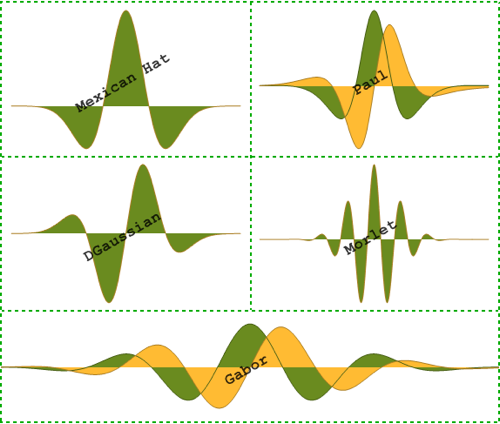Wavelets themselves are short-lived wave-like oscillations. Taking the Morlet wavelet, for example, we can see that unlike sines and cosines, this wave-like oscillation is localized in the sense that it does not stretch out to infinity.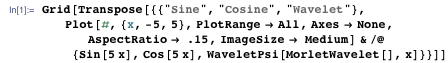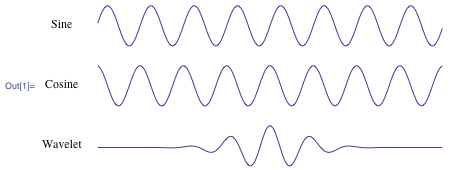When applied to time-series data, wavelet analysis involves a transform from the given one-dimensional time series to a two-dimensional time-frequency image. This wavelet transform utilizes a basic function (called the mother wavelet), then dilates (scaling) and translates (translation) it to capture features that are local in time and frequency. Because of this localization, wavelets are useful when analyzing signals with sharp discontinuities.

Returning to the Morlet wavelet, we can use the following demonstration to show how the scaling and translation is accomplished.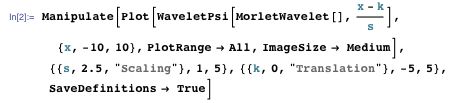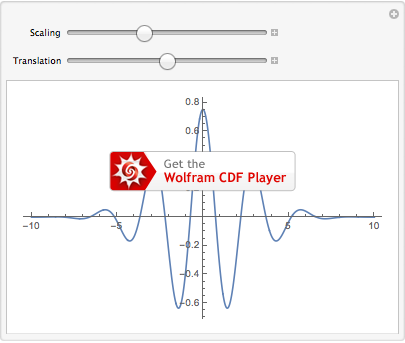If the scale parameter increases, then the wavelet function is stretched in the time domain, and therefore shrunk in the frequency domain. Because of scaling, we can then process data at different resolutions. We can use a large scale (lower frequencies) for signals with large features and a small scale for small features. The rescaled wavelets together form a wavelet family or basis.

Translation allows wavelets to be translated along the time-axis of the time-series data, so that the times when certain features appear can be detected.

One large usage of wavelets, and of smoothing functions in general, is image compression. If you’ve ever looked at a JPEG 2000 image, then you’ve seen smoothing functions in action. With wavelets, a given image is put through wavelet smoothing multiple times, and then all small values are thrown out. This lossy method can save a lot of space, sometimes as much as 99%. When the FBI wanted to make digital versions of 30 million fingerprints, they used WSQ (Wavelet Scalar Quantization) to cut storage costs by a factor of more than 10.

To demonstrate how wavelets are used in image compression, we take one famous image in fractals called the Mandelbrot set. It is known for its elaborate boundary that reveals ever finer recursive details in increasing magnification.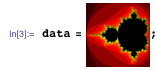To perform an image compression, we need to first apply a discrete wavelet transform to the image. A discrete wavelet transform decomposes the given image into an approximate space (low frequency components) and detail space (high frequency components). The wavelet coefficients are obtained from projecting portions of the image with translated and dilated versions of the wavelet function.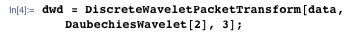In order to compare the effect of differing amounts of compression, we need to choose a set of threshold values (δ) and perform wavelet compression for each value.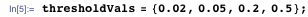Using WaveletBestBasis, Mathematica selects an optimal basis to be used given the threshold values.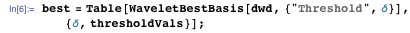To perform thresholding, we wrap WaveletThreshold around the optimal basis. The threshold value δ will stay the same for both the optimal basis and thresholding.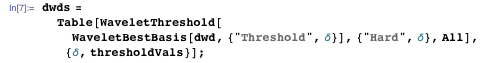To perform the image compression, we only need to keep the nonzero terms. Therefore, we can compare the nonzero values in the original data to the thresholded coefficients as a measure of compression.

The number of nonzeros in the original image is: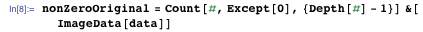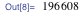Note the third argument in Count uses the command Depth to ensure that the nonzero items at the appropriate depth are counted.

The number of nonzeros in the compressed image with a threshold value of 0.02 is: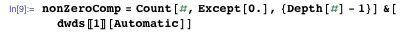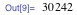The compression measure is therefore: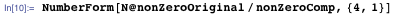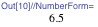Thus, the amount of data required to store the image has been reduced by a factor of 6.5.

By placing half of the original image on top of the reconstructed image, we can compare them and obtain the compression measure for all the threshold values.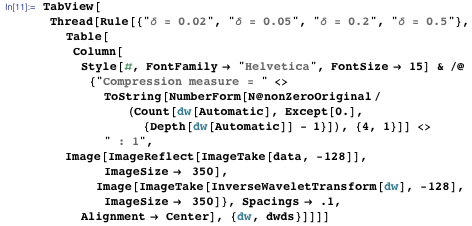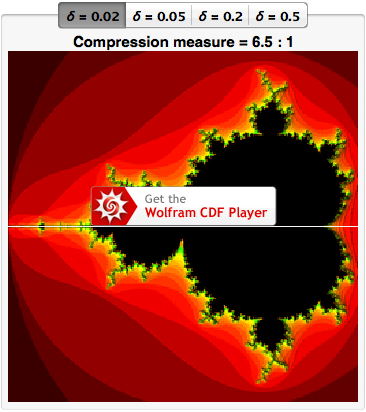At a threshold value of 0.02, the difference between the original and compressed image might be hard to tell with the naked eye. But, with any lossy compression, there must be a difference. But how much difference? We can use ImageDifference to extract that information.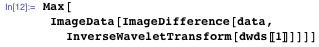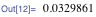So the difference between these two images is a maximum of 3% in pixel value. No wonder it’s hard to see!

Wavelet analysis can be used in many other application areas. Signal detection in time-series data is one natural extension of wavelets. Keep an eye out for another post on useful applications of wavelets.

To learn more about working with wavelets in Mathematica, watch our Wolfram Training on-demand courses on Wavelet Analysis.